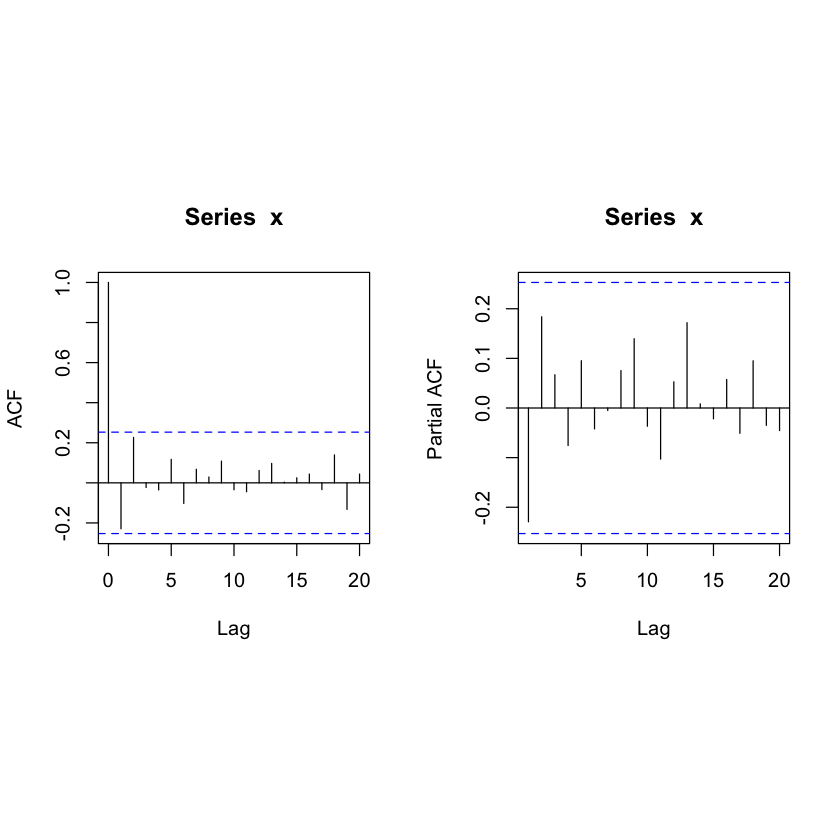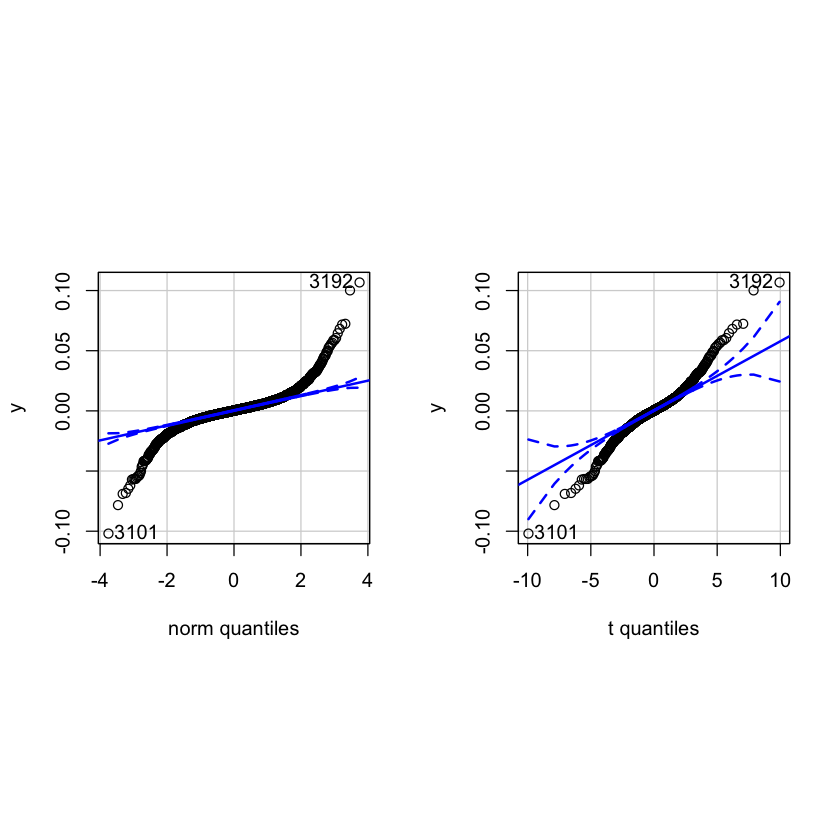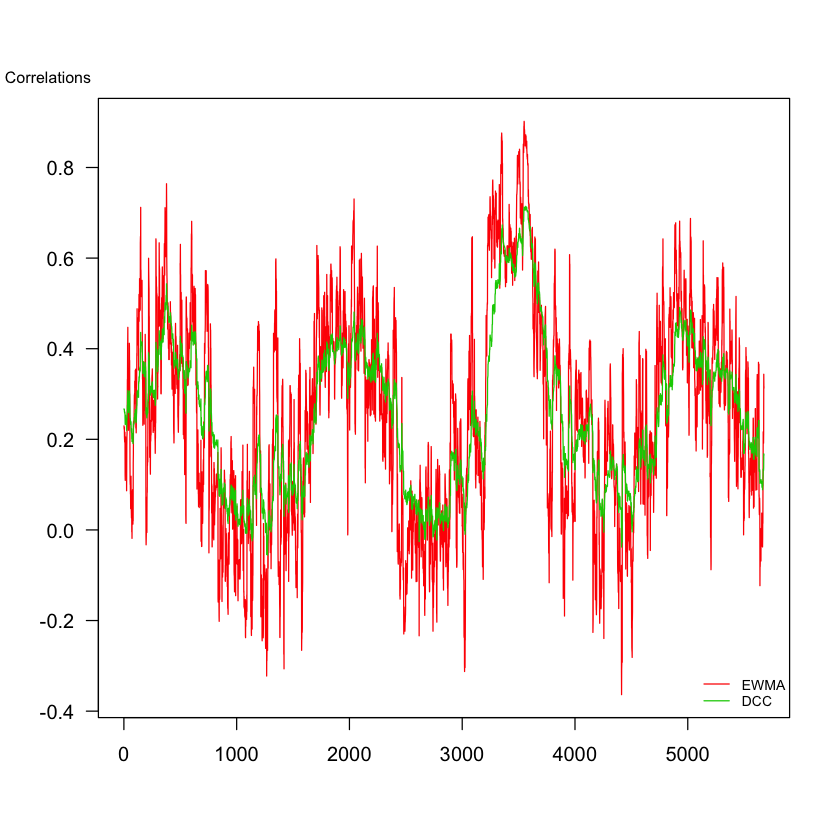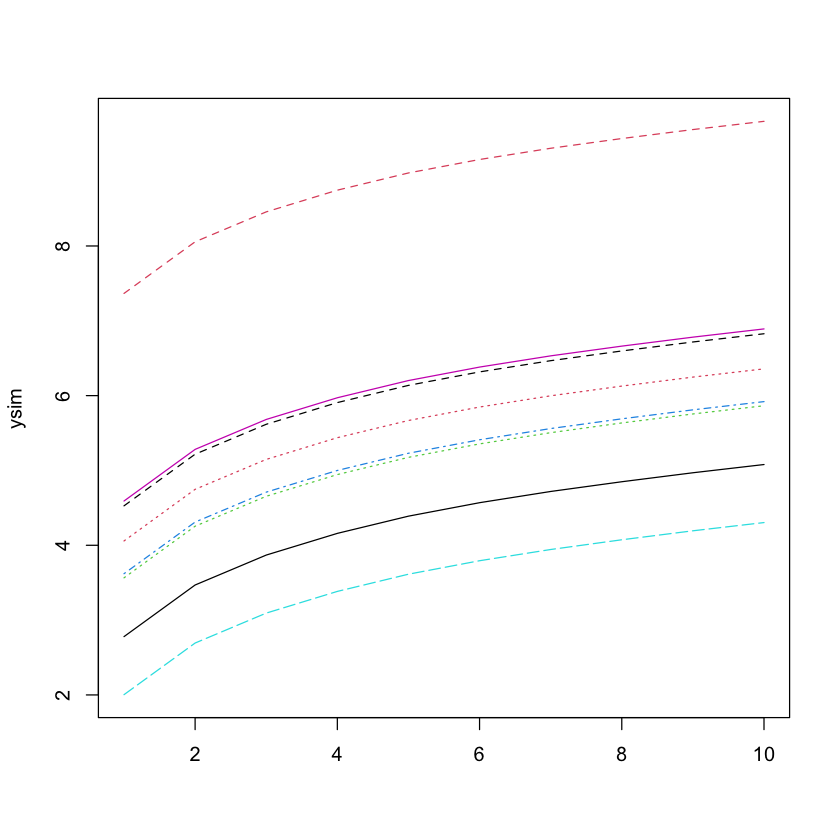Introduction¶

This notebook is an implementation of Jón Daníelsson's Financial Risk Forecasting (Wiley, 2011) in R 4.0, with annotations and introductory examples. The introductory examples (Appendix) are similar to Appendix B in the original book.

Bullet point numbers correspond to the R/MATLAB Listing numbers in the original book, referred to henceforth as FRF.

More details can be found at the book website: https://www.financialriskforecasting.com/

Last updated: July 2020

Copyright 2011- 2020 Jón Daníelsson. This code is free software: you can redistribute it and/or modify it under the terms of the GNU General Public License as published by the Free Software Foundation, either version 3 of the License, or (at your option) any later version. This code is distributed in the hope that it will be useful, but WITHOUT ANY WARRANTY; without even the implied warranty of MERCHANTABILITY or FITNESS FOR A PARTICULAR PURPOSE. See the GNU General Public License for more details. The GNU General Public License is available at: https://www.gnu.org/licenses/.

Appendix: An Introduction to R¶

Created in R 4.0 (July 2020)

• R.1: Entering and Printing Data
• R.2: Vectors, Matrices and Sequences
• R.3: Importing Data (to be updated)
• R.4: Basic Summary Statistics
• R.5: Calculating Moments
• R.6: Basic Matrix Operations
• R.7: Statistical Distributions
• R.8: Statistical Tests
• R.9: Time Series
• R.10: Loops and Functions
• R.11: Basic Graphs
• R.12: Miscellaneous Useful Functions
In :
# Entering and Printing Data in R
# Listing R.1
# Last updated June 2018
#
#

x = 10             # assign x the value 10
print(x)           # print x
 10
In :
# Vectors, Matrices and Sequences in R
# Listing R.2
# Last updated June 2018
#
#

y = c(1,3,5,7,9)          # create vector using c()

print(y)

print(y)               # calling 3rd element (R indices start at 1)

print(dim(y))             # gives NULL since y is a vector, not a matrix

print(length(y))          # as expected, y has length 5

v = matrix(nrow=2,ncol=3) # fill a 2 x 3 matrix with NaN values (default)

print(dim(v))             # as expected, v is size (2,3)

w = matrix(c(1,2,3),nrow=6,ncol=3) # repeats matrix twice by rows, thrice by columns

print(w)

s = 1:10                  # s is a list of integers from 1 to 10 inclusive

print(s)
 1 3 5 7 9
 5
NULL
 5
 2 3
[,1] [,2] [,3]
[1,]    1    1    1
[2,]    2    2    2
[3,]    3    3    3
[4,]    1    1    1
[5,]    2    2    2
[6,]    3    3    3
  1  2  3  4  5  6  7  8  9 10
In :
# Basic Summary Statistics in R
# Listing R.3
# Last updated June 2018
#
#

y=matrix(c(3.1,4.15,9))

sum(y)         # sum of all elements of y
prod(y)        # product of all elements of y
max(y)         # maximum value of y
min(y)         # minimum value of y
range(y)       # min, max value of y
mean(y)        # arithmetic mean
median(y)      # median
var(y)         # variance
cov(y)         # covar matrix = variance for single vector
cor(y)         # corr matrix =  for single vector
sort(y)        # sorting in ascending order
log(y)         # natural log
16.25
115.785
9
3.1
1. 3.1
2. 9
5.41666666666667
4.15
 9.90583
 9.90583
 1
1. 3.1
2. 4.15
3. 9
 1.1314 1.42311 2.19722
In :
# Calculating Moments in R
# Listing R.4
# Last updated June 2018
#
#

library(moments)

mean(y)      # mean
var(y)       # variance
sd(y)        # unbiased standard deviation, by default
skewness(y)  # skewness
kurtosis(y)  # kurtosis
5.41666666666667
 9.90583
3.14735338551827
0.61960293299089
1.5
In :
# Basic Matrix Operations in R
# Listing R.5
# Last updated June 2018
#
#

z = matrix(c(1,2,3,4),2,2)   # z is a 2 x 2 matrix
x = matrix(c(1,2),1,2)       # x is a 1 x 2 matrix

## Note: z * x is undefined since the two matrices are not conformable

z %*% t(x)                   # this evaluates to a 2 x 1 matrix

rbind(z,x)                   # "stacking" z and x vertically
cbind(z,t(x))                # "stacking z and x' horizontally

## Note: dimensions must match along the combining axis
 7 10
 1 3 2 4 1 2
 1 3 1 2 4 2
In :
# Statistical Distributions in R
# Listing R.6
# Last updated June 2018
#
#

q = seq(from = -3, to = 3, length = 7)     # specify a set of values

p = seq(from = 0.1, to = 0.9, length = 9)  # specify a set of probabilities

qnorm(p, mean = 0, sd = 1)                 # element-wise inverse Normal quantile

pt(q, df = 4)                              # element-wise cdf under Student-t(4)

dchisq(q, df = 2)                          # element-wise pdf under Chisq(2)

## Similar syntax for other distributions
## q for quantile, p for cdf, d for pdf
## followed by the abbreviation of the distribution

## One can also obtain pseudorandom samples from distributions

x = rt(100, df = 5)                        # Sampling 100 times from TDist with 5 df

y = rnorm(50, mean = 0, sd = 1)            # Sampling 50 times from a standard normal

## Given data, we obtain MLE estimates of distribution parameters with package MASS:

library(MASS)

res = fitdistr(x, densfun = "normal")      # Fitting x to normal dist

print(res)
1. -1.2815515655446
2. -0.841621233572914
3. -0.524400512708041
4. -0.2533471031358
5. 0
6. 0.2533471031358
7. 0.524400512708041
8. 0.841621233572914
9. 1.2815515655446
1. 0.0199709840358594
2. 0.0580582617584078
3. 0.186950483150029
4. 0.5
5. 0.813049516849971
6. 0.941941738241592
7. 0.980029015964141
1. 0
2. 0
3. 0
4. 0.5
5. 0.303265329856317
6. 0.183939720585721
7. 0.111565080074215
mean           sd
-0.18367897    1.23654134
( 0.12365413) ( 0.08743668)
In :
# Statistical Tests in R
# Listing R.7
# Last updated June 2018
#
#

library(tseries)

x = rt(500, df = 5)                            # Create hypothetical dataset x

jarque.bera.test(x)                            # Jarque-Bera test for normality
Box.test(x, lag = 20, type = c("Ljung-Box"))   # Ljung-Box test for serial correlation
Jarque Bera Test

data:  x
X-squared = 279.82, df = 2, p-value < 2.2e-16
Box-Ljung test

data:  x
X-squared = 16.548, df = 20, p-value = 0.6821
In :
# Time Series in R
# Listing R.8
# Last updated June 2018
#
#

x = rt(60, df = 5)  # Create hypothetical dataset x

par(mfrow=c(1,2), pty='s')

acf(x,20)           # autocorrelation for lags 1:20
pacf(x,20)          # partial autocorrelation for lags 1:20In :
# Loops and Functions in R
# Listing R.9
# Last updated June 2018
#
#

## For loops

for (i in 3:7)        # iterates through [3,4,5,6,7]
print(i^2)

## If-else loops

X = 10

if (X %% 3 == 0) {
print("X is a multiple of 3")
} else {
print("X is not a multiple of 3")
}

## Functions (example: a simple excess kurtosis function)

excess_kurtosis = function(x, excess = 3){ # note: excess optional, default=3
m4 = mean((x-mean(x))^4)
excess_kurt = m4/(sd(x)^4) - excess
excess_kurt
}

x = rt(60, df = 5)                         # Create hypothetical dataset x

excess_kurtosis(x)
 9
 16
 25
 36
 49
 "X is not a multiple of 3"
-0.278679706252703
In :
# Basic Graphs in R
# Listing R.10
# Last updated June 2018
#
#

y = rnorm(50, mean = 0, sd = 1)

par(mfrow=c(2,2)) # sets up space for subplots

barplot(y)        # bar plot
plot(y,type='l')  # line plot
hist(y)           # histogram
plot(y)           # scatter plotIn :
# Miscellaneous Useful Functions in R
# Listing R.11
# Last updated June 2018
#
#

## Convert objects from one type to another with as.integer() etc
## To check type, use typeof(object)

x = 8.0

print(typeof(x))

x = as.integer(x)

print(typeof(x))
 "double"
 "integer"

Chapter 1: Financial Markets, Prices and Risk¶

• 1.3: Summary statistics for returns timeseries
• 1.5: Autocorrelation function (ACF) plots, Ljung-Box test
• 1.7: Quantile-Quantile (QQ) plots
• 1.9: Correlation matrix between different stocks
In :
# Listing 1.1
# Last updated August 2019
#
#

y=diff(log(price$Index)) # calculate returns plot(y) # plot returnsIn : # Sample statistics in R # Listing 1.3 # Last updated July 2020 # # library(moments) library(tseries) mean(y) sd(y) min(y) max(y) skewness(y) kurtosis(y) jarque.bera.test(y) 0.000258159900825998 0.0100057286437631 -0.101955486273023 0.106735895029117 0.152633269896337 16.981171461092 Jarque Bera Test data: y X-squared = 46251, df = 2, p-value < 2.2e-16 In : # ACF plots and the Ljung-Box test in R # Listing 1.5 # Last updated July 2020 # # library(MASS) library(stats) par(mfrow=c(1,2), pty="s") q = acf(y,20) q1 = acf(y^2,20) Box.test(y, lag = 20, type = c("Ljung-Box")) Box.test(y^2, lag = 20, type = c("Ljung-Box")) Box-Ljung test data: y X-squared = 93.488, df = 20, p-value = 1.809e-11 Box-Ljung test data: y^2 X-squared = 2543, df = 20, p-value < 2.2e-16In : # QQ plots in R # Listing 1.7 # Last updated June 2018 # # library(car) par(mfrow=c(1,2), pty="s") qqPlot(y) qqPlot(y,distribution="t",df=5) 1. 3192 2. 3101 1. 3192 2. 3101In : # Download stock prices in R # Listing 1.9 # Last updated June 2018 # # p = read.csv('stocks.csv') y=apply(log(p),2,diff) print(cor(y)) # correlation matrix A B C A 1.0000000 0.2296842 0.2126192 B 0.2296842 1.0000000 0.1450511 C 0.2126192 0.1450511 1.0000000 Chapter 2: Univariate Volatility Modelling¶ • 2.1: GARCH and t-GARCH estimation • 2.3: APARCH estimation In : # ARCH and GARCH estimation in R # Listing 2.1 # Last updated July 2020 # # library(rugarch) p = read.csv('index.csv') ## We multiply returns by 100 and de-mean them y=diff(log(p$Index))*100
y=y-mean(y)

## GARCH(1,1)
spec1 = ugarchspec(variance.model = list( garchOrder = c(1, 1)),
mean.model = list( armaOrder = c(0,0),include.mean = FALSE))
res1 = ugarchfit(spec = spec1, data = y)

## ARCH(1)
spec2 = ugarchspec(variance.model = list( garchOrder = c(1, 0)),
mean.model = list( armaOrder = c(0,0),include.mean = FALSE))
res2 = ugarchfit(spec = spec2, data = y)

## tGARCH(1,1)
spec3 = ugarchspec(variance.model = list( garchOrder = c(1, 1)),
mean.model = list( armaOrder = c(0,0),include.mean = FALSE),
distribution.model = "std")
res3 = ugarchfit(spec = spec3, data = y)

## plot(res) shows various graphical analysis, works in command line
In :
# Advanced ARCH and GARCH estimation in R
# Listing 2.3
# Last updated July 2020
#
#

## Normal APARCH(1,1)
spec4 = ugarchspec(variance.model = list(model="apARCH", garchOrder = c(1, 1)),
mean.model = list( armaOrder = c(0,0),include.mean = FALSE))
res4 = ugarchfit(spec = spec4, data = y)
## show(res4)

## Normal APARCH(1,1) with fixed delta
spec5 = ugarchspec(variance.model = list(model="apARCH", garchOrder = c(1, 1)),
mean.model = list( armaOrder = c(0,0),include.mean = FALSE), fixed.pars=list(delta=2))
res5 = ugarchfit(spec = spec5, data = y)
show(res5)
*---------------------------------*
*          GARCH Model Fit        *
*---------------------------------*

Conditional Variance Dynamics
-----------------------------------
GARCH Model	: apARCH(1,1)
Mean Model	: ARFIMA(0,0,0)
Distribution	: norm

Optimal Parameters
------------------------------------
Estimate  Std. Error   t value Pr(>|t|)
omega   0.013024    0.001742   7.47834  0.00000
alpha1  0.068106    0.005309  12.82896  0.00000
beta1   0.918173    0.005618 163.42376  0.00000
gamma1  0.009355    0.031721   0.29493  0.76804
delta   2.000000          NA        NA       NA

Robust Standard Errors:
Estimate  Std. Error  t value Pr(>|t|)
omega   0.013024    0.003600  3.61724 0.000298
alpha1  0.068106    0.009486  7.17951 0.000000
beta1   0.918173    0.012216 75.16415 0.000000
gamma1  0.009355    0.060856  0.15373 0.877821
delta   2.000000          NA       NA       NA

LogLikelihood : -6970.28

Information Criteria
------------------------------------

Akaike       2.4575
Bayes        2.4621
Shibata      2.4575
Hannan-Quinn 2.4591

Weighted Ljung-Box Test on Standardized Residuals
------------------------------------
statistic p-value
Lag                      3.616 0.05722
Lag[2*(p+q)+(p+q)-1]     3.857 0.08240
Lag[4*(p+q)+(p+q)-1]     4.221 0.22779
d.o.f=0
H0 : No serial correlation

Weighted Ljung-Box Test on Standardized Squared Residuals
------------------------------------
statistic p-value
Lag                     0.4164  0.5188
Lag[2*(p+q)+(p+q)-1]    3.3472  0.3473
Lag[4*(p+q)+(p+q)-1]    4.2909  0.5403
d.o.f=2

Weighted ARCH LM Tests
------------------------------------
Statistic Shape Scale P-Value
ARCH Lag    0.5263 0.500 2.000  0.4682
ARCH Lag    0.5995 1.440 1.667  0.8539
ARCH Lag    0.9614 2.315 1.543  0.9198

Nyblom stability test
------------------------------------
Joint Statistic:  0.5702
Individual Statistics:
omega  0.12447
alpha1 0.22152
beta1  0.14319
gamma1 0.08482

Asymptotic Critical Values (10% 5% 1%)
Joint Statistic:     	 1.07 1.24 1.6
Individual Statistic:	 0.35 0.47 0.75

Sign Bias Test
------------------------------------
t-value   prob sig
Sign Bias          1.10613 0.2687
Negative Sign Bias 0.22448 0.8224
Positive Sign Bias 0.06151 0.9510
Joint Effect       1.66247 0.6453

------------------------------------
group statistic p-value(g-1)
1    20     308.5    3.656e-54
2    30     353.5    1.753e-57
3    40     381.3    9.740e-58
4    50     393.5    2.611e-55

Elapsed time : 0.3847032

Chapter 3: Multivariate Volatility Models¶

• 3.3: EWMA estimation
• 3.5: OGARCH estimation
• 3.7: DCC estimation
• 3.9: Comparison of EWMA, OGARCH, DCC
In :
# Listing 3.1
# Last updated August 2019
#
#

y=apply(log(p),2,diff)     # calculate returns
y = y[,1:2]                # consider first two stocks
y[,1] = y[,1]-mean(y[,1])  # subtract mean
y[,2] = y[,2]-mean(y[,2])
TT = dim(y)
In :
# EWMA in R
# Listing 3.3
# Last updated August 2019
#
#

## create a matrix to hold covariance matrix for each t
EWMA = matrix(nrow=TT,ncol=3)
lambda = 0.94
S = cov(y)                      # initial (t=1) covar matrix
EWMA[1,] = c(S)[c(1,4,2)]       # extract var and covar
for (i in 2:dim(y)){
S = lambda*S+(1-lambda)*  y[i-1,] %*% t(y[i-1,])
EWMA[i,] = c(S)[c(1,4,2)]
}
EWMArho = EWMA[,3]/sqrt(EWMA[,1]*EWMA[,2]) # calculate correlations

print(tail(EWMArho))
 0.2296842 0.2236347 0.2218277 0.2270681 0.2054204 0.1992979
 0.1931994 0.1914987 0.2177147 0.3436632 0.3122741 0.3159703
In :
# GOGARCH in R
# Listing 3.5
# Last updated August 2019
#
#

library(rmgarch)
spec = gogarchspec(mean.model = list(armaOrder = c(0, 0),
include.mean =FALSE),
variance.model = list(model = "sGARCH",
garchOrder = c(1,1)) ,
distribution.model =  "mvnorm"
)
fit = gogarchfit(spec = spec, data = y)

show(fit)
*------------------------------*
*        GO-GARCH Fit          *
*------------------------------*

Mean Model		: CONSTANT
GARCH Model		: sGARCH
Distribution	: mvnorm
ICA Method		: fastica
No. Factors		: 2
No. Periods		: 5676
Log-Likelihood	: 40378.57
------------------------------------

U (rotation matrix) :

[,1]  [,2]
[1,] -0.358 0.934
[2,]  0.934 0.358

A (mixing matrix) :

[,1]     [,2]
[1,] -0.00642 0.000213
[2,] -0.00187 0.009296

In :
# DCC in R
# Listing 3.7
# Last updated August 2019
#
#

xspec = ugarchspec(mean.model = list(armaOrder = c(0, 0), include.mean = FALSE))

uspec = multispec(replicate(2, xspec))

spec = dccspec(uspec = uspec, dccOrder = c(1, 1), distribution = 'mvnorm')

res = dccfit(spec, data = y)

H=res@mfit$H DCCrho=vector(length=dim(y)) for(i in 1:dim(y)){ DCCrho[i] = H[1,2,i]/sqrt(H[1,1,i]*H[2,2,i]) } In : # Sample statistics in R # Listing 3.9 # Last updated August 2019 # # matplot(cbind(EWMArho,DCCrho),type='l',las=1,lty=1,col=2:3,ylab="") mtext("Correlations",side=2,line=0.3,at=1,las=1,cex=0.8) legend("bottomright",c("EWMA","DCC"),lty=1,col=2:3,bty="n",cex=0.7)Chapter 4: Risk Measures¶ • 4.1: Expected Shortfall (ES) estimation under normality assumption In : # ES in R # Listing 4.1 # Last updated August 2016 # # p = c(0.5,0.1,0.05,0.025,0.01,0.001) VaR = -qnorm(p) ES = dnorm(qnorm(p))/p cat("Probabilities:", paste0(p*100,"%"), "\n", "VaR:", VaR, "\n", "ES:", ES) Probabilities: 50% 10% 5% 2.5% 1% 0.1% VaR: 0 1.281552 1.644854 1.959964 2.326348 3.090232 ES: 0.7978846 1.754983 2.062713 2.337803 2.665214 3.36709 Chapter 5: Implementing Risk Forecasts¶ • 5.1: Loading hypothetical stock prices, converting to returns • 5.3: Univariate HS Value at Risk (VaR) • 5.5: Multivariate HS VaR • 5.7: Univariate ES VaR • 5.9: Normal VaR • 5.11: Portfolio Normal VaR • 5.13: Student-t VaR • 5.15: Normal ES VaR • 5.17: Direct Integration Normal ES VaR • 5.19: MA Normal VaR • 5.21: EWMA VaR • 5.23: Two-asset EWMA VaR • 5.25: GARCH(1,1) VaR In : # Download stock prices in R # Listing 5.1 # Last updated July 2020 # # p = read.csv('stocks.csv') ## Calculate returns. Note that first column is dates y = apply(log(p),2,diff) ## Specify portfolio value and VaR probability portfolio_value = 1000 p = 0.01 In : # Univariate HS in R # Listing 5.3 # Last updated July 2020 # # y1 = y[,1] # select one asset ys = sort(y1) # sort returns op = ceiling(length(y1)*p) # p percent smallest, rounded up VaR1 = -ys[op]*portfolio_value print(VaR1)  17.49822 In : # Multivariate HS in R # Listing 5.5 # Last updated July 2020 # # w = matrix(c(0.3,0.2,0.5)) # vector of portfolio weights ## The number of columns of the left matrix must be the same as the number of rows of the right matrix yp = y %*% w # obtain portfolio returns yps = sort(yp) VaR2 = -yps[op]*portfolio_value print(VaR2)  14.20901 In : # Univariate ES in R # Listing 5.7 # Last updated August 2019 # # ES1 = -mean(ys[1:op])*portfolio_value print(ES1)  22.56339 In : # Normal VaR in R # Listing 5.9 # Last updated August 2019 # # sigma = sd(y1) # estimate volatility VaR3 = -sigma * qnorm(p) * portfolio_value print(VaR3)  14.94957 In : # Portfolio normal VaR in R # Listing 5.11 # Last updated August 2019 # # sigma = sqrt(t(w) %*% cov(y) %*% w) # portfolio volatility ## Note: the trailing  is to convert a single element matrix to float VaR4 = -sigma * qnorm(p)*portfolio_value print(VaR4)  12.27959 In : # Student-t VaR in R # Listing 5.13 # Last updated July 2020 # # library(QRM) scy1 = (y1)*100 # scale the returns res = fit.st(scy1) sigma1 = unname(res$par.ests['sigma']/100)  # rescale the volatility
nu = unname(res$par.ests['nu']) ## Note: We are removing the names of the fit.st output using unname() VaR5 = - sigma1 * qt(df=nu,p=p) * portfolio_value print(VaR5)  17.12558 In : # Normal ES in R # Listing 5.15 # Last updated June August 2019 # # sigma = sd(y1) ES2 = sigma*dnorm(qnorm(p))/p * portfolio_value print(ES2)  17.12719 In : # Direct integration ES in R # Listing 5.17 # Last updated July 2020 # # VaR = -qnorm(p) integrand = function(q){q*dnorm(q)} ES = -sigma*integrate(integrand,-Inf,-VaR)$value/p*portfolio_value

print(ES)
 17.12719
In :
# MA normal VaR in R
# Listing 5.19
# Last updated June August 2019
#
#

WE=20
for (t in seq(length(y1)-5,length(y1))){
t1=t-WE+1
window= y1[t1:t] # estimation window
sigma=sd(window)
VaR6 = -sigma * qnorm(p) * portfolio_value
print(VaR6)
}
 16.0505
 16.1491
 18.85435
 18.88212
 16.23053
 16.16976
In :
# EWMA VaR in R
# Listing 5.21
# Last updated July 2020
#
#

lambda = 0.94
s11 = var(y1)             # initial variance, using unconditional
for (t in 2:length(y1)){
s11 = lambda * s11  + (1-lambda) * y1[t-1]^2
}
VaR7 = -qnorm(p) * sqrt(s11) * portfolio_value

print(VaR7)
 16.75344
In :
# Three-asset EWMA VaR in R
# Listing 5.23
# Last updated August 2019
#
#

s = cov(y)                        # initial covariance
for (t in 2:dim(y)){
s = lambda*s + (1-lambda)*y[t-1,] %*% t(y[t-1,])
}
sigma = sqrt(t(w) %*% s %*% w) # portfolio vol
## Note: trailing is to convert single element matrix to float

VaR8 = -sigma * qnorm(p) * portfolio_value

print(VaR8)
 11.50952
In :
# Univariate GARCH in R
# Listing 5.25
# Last updated July 2020
#
#
library(rugarch)

spec = ugarchspec(variance.model = list( garchOrder = c(1, 1)),
mean.model = list( armaOrder = c(0,0),include.mean = FALSE))
res = ugarchfit(spec = spec, data = y1)

omega = res@fit$coef['omega'] alpha = res@fit$coef['alpha1']
beta = res@fit$coef['beta1'] sigma2 = omega + alpha * tail(y1,1)^2 + beta * tail(res@fit$var,1)
VaR9 = -sqrt(sigma2) * qnorm(p) * portfolio_value
names(VaR9)="VaR"
print(VaR9)
VaR
16.88628

Chapter 6: Analytical Value-at-Risk for Options and Bonds¶

• 6.1: Black-Scholes function definition
• 6.3: Black-Scholes option price calculation example
In :
# Black-Scholes function in R
# Listing 6.1
# Last updated 2011
#
#

bs = function(X, P, r, sigma, T){
d1 = (log(P/X) + (r + 0.5*sigma^2)*(T))/(sigma*sqrt(T))
d2 = d1 - sigma*sqrt(T)

Call = P*pnorm(d1,mean=0,sd=1)-X*exp(-r*(T))*pnorm(d2,mean=0,sd=1)
Put = X*exp(-r*(T))*pnorm(-d2,mean=0,sd=1)-P*pnorm(-d1,mean=0,sd=1)

Delta.Call = pnorm(d1, mean = 0, sd = 1)
Delta.Put = Delta.Call - 1
Gamma = dnorm(d1, mean = 0, sd = 1)/(P*sigma*sqrt(T))

return(list(Call=Call,Put=Put,Delta.Call=Delta.Call,Delta.Put=Delta.Put,Gamma=Gamma))
}
In :
# Black-Scholes in R
# Listing 6.3
# Last updated July 2020
#
#

f = bs(X = 90, P = 100, r = 0.05, sigma = 0.2, T = 0.5)

print(f)
$Call  13.49852$Put
 1.27641

$Delta.Call  0.8395228$Delta.Put
 -0.1604772

\$Gamma
 0.01723826

Chapter 7: Simulation Methods for VaR for Options and Bonds¶

• 7.1: Plotting normal distribution transformation
• 7.3: Random number generation from Uniform(0,1), Normal(0,1)
• 7.5: Bond pricing using yield curve
• 7.7: Yield curve simulations
• 7.9: Bond price simulations
• 7.11: Black-Scholes analytical pricing of call
• 7.13: Black-Scholes Monte Carlo simulation pricing of call
• 7.15: Option density plots
• 7.17: VaR simulation of portfolio with only underlying
• 7.19: VaR simulation of portfolio with only call
• 7.21: VaR simulation of portfolio with call, put and underlying
• 7.23: Simulated two-asset returns
• 7.25: Two-asset portfolio VaR
• 7.27: Two-asset portfolio VaR with a call
In :
# Transformation in R
# Listing 7.1
# Last updated 2011
#
#

x = seq(-3, 3, by = 0.1)
plot(x, pnorm(x), type = "l")In :
# Various RNs in R
# Listing 7.3
# Last updated 2011
#
#

set.seed(12) # set seed

S = 10
runif(S)
rnorm(S)
rt(S,4)
1. 0.0693609158042818
2. 0.817775198724121
3. 0.942621732363477
4. 0.269381876336411
5. 0.169348123250529
6. 0.0338956224732101
7. 0.178785004187375
8. 0.641665365546942
9. 0.0228777434676886
10. 0.00832482660189271
1. -0.27229604424923
2. -0.315348711467784
3. -0.628255236517538
4. -0.106463884872094
5. 0.428014802202354
6. -0.777719582147445
7. -1.29388229761362
8. -0.779566508059429
9. 0.0119517585652984
10. -0.152416237822168
1. -0.54686521181825
2. 0.325766227984669
3. -0.310344756918247
4. 1.64011963529838
5. -0.60065788608405
6. -0.504397302337928
7. 0.146674867812759
8. 0.421606955584244
9. -1.10969020069546
10. 0.674102188748512
In :
# Price bond in R
# Listing 7.5
# Last updated July 2020
#
#

yield = c(5.00, 5.69, 6.09, 6.38, 6.61,
6.79, 6.94, 7.07, 7.19, 7.30)   # yield curve
T = length(yield)                       # number of time periods
r = 0.07                                # initial yield rate
Par = 10                                # par value
coupon = r * Par                        # coupon payments
cc = rep(coupon, T)                     # vector of cash flows
cc[T] = cc[T] + Par                     # add par to cash flows
P = sum(cc/((1+yield/100)^(1:T)))      # calculate price

print(P)
 9.913206
In :
# Simulate yields in R
# Listing 7.7
# Last updated August 2019
#
#

set.seed(12)                           # set seed

sigma = 1.5                            # daily yield volatiltiy
S = 8                                  # number of simulations
r = rnorm(S, 0, sigma)                 # generate random numbers
ysim = matrix(nrow=length(yield),ncol=S)
for (i in 1:S) ysim[,i]=yield+r[i]
matplot(ysim,type='l')In :
# Simulate bond prices in R
# Listing 7.9
# Last updated November 2020
#
#

SP = rep(NA, length = S)
for (i in 1:S){                            # S simulations
SP[i] = sum(cc/((1+ysim[,i]/100)^(1:T)))
}
SP = SP-(mean(SP) - P)                     # correct for mean

par(mfrow=c(1,2), pty="s")

barplot(SP)

hist(SP,probability=TRUE)
x=seq(6,16,length=100)
lines(x, dnorm(x, mean = mean(SP), sd = sd(SP)))

S = 50000
r = rnorm(S, 0, sigma)                 # generate random numbers
ysim = matrix(nrow=length(yield),ncol=S)
for (i in 1:S) ysim[,i]=yield+r[i]

SP = rep(NA, length = S)
for (i in 1:S){                            # S simulations
SP[i] = sum(cc/((1+ysim[,i]/100)^(1:T)))
}
SP = SP-(mean(SP) - P)                     # correct for mean

par(mfrow=c(1,2), pty="s")

barplot(SP)

hist(SP,probability=TRUE)
x=seq(6,16,length=100)
lines(x, dnorm(x, mean = mean(SP), sd = sd(SP)))